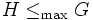# Maximal subgroup

VIEW: Definitions built on this | Facts about this: (facts closely related to Maximal subgroup, all facts related to Maximal subgroup) |Survey articles about this | Survey articles about definitions built on this
VIEW RELATED: Analogues of this | Variations of this | Opposites of this |[SHOW MORE]
This article defines a subgroup property: a property that can be evaluated to true/false given a group and a subgroup thereof, invariant under subgroup equivalence. View a complete list of subgroup properties[SHOW MORE]

## History

The notion of maximal subgroup probably dates back to the very beginning of group theory.

## Definition

### Symbol-free definition

A maximal subgroup of a group is defined in the following equivalent ways:

### Definition with symbols

A subgroup$H$ of a group$G$ is termed maximal (in symbols,$H \le_{\max} G$ or$G \ge_{\max} H$Notations) if it satisfies the following equivalent condition:

•$H$ is a proper subgroup of$G$ (i.e.$H \ne G$) and if$H \le K \le G$ for some subgroup$K$, then$H = K$ or$K = G$.
•$H$ is proper and the action of$G$ on the coset space$G/H$ is a primitive group action: there is no nontrivial partition of the coset space into blocks such that$G$ preserves the partition.

### In terms of group actions

In terms of group actions, a subgroup of a group is maximal if the natural group action on its coset space is primitive.

## Formalisms

### Monadic second-order description

This subgroup property is a monadic second-order subgroup property, viz., it has a monadic second-order description in the theory of groups
View other monadic second-order subgroup properties

The property of being a maximal subgroup can be expressed in monadic second-order logic: there is no bigger subgroup between the given subgroup and the whole group.

### In terms of the maximal operator

This property is obtained by applying the maximal operator to the property: proper subgroup
View other properties obtained by applying the maximal operator

## Relation with other properties

### Property bifurcations

There are many pairs of properties such that every maximal subgroup of a group has exactly one of these properties. For a complete list, refer:

## Metaproperties

### Transfer condition

In general, it may not be true that the intersection of a maximal subgroup with another subgroup is maximal inside that subgroup. If a subgroup has the property that its intersection with every maximal subgroup (not containing it) is maximal in it, the subgroup is termed max-sensitive.

## Property operators

### Transiters

The left and right transiters are both the identity element.

### Subordination

The subordination property on the property of maximality defines the property of submaximality. For finite groups, every subgroup is submaximal. However, this may not be true in general for infinite groups. It is, however, true that every subgroup of finite index is submaximal.

### The maximal operator

The maximal operator is a subgroup property modifier that takes any subgroup property and gives out the property of being a subgroup that is maximal in the group with respect to that property.

## Testing

### GAP command

This subgroup property can be tested using built-in functionality of Groups, Algorithms, Programming (GAP).
The GAP command for listing all subgroups with this property is:MaximalSubgroups
The GAP command for listing all conjugacy classes of subgroups with this property is:ConjugacyClassesMaximalSubgroups
The GAP command for listing a representative of each conjugacy class of subgroups with this property is:MaximalSubgroupClassReps
View subgroup properties testable with built-in GAP command|View subgroup properties for which all subgroups can be listed with built-in GAP commands | View subgroup properties codable in GAP